Courses

# Test: Lines And Angles - 3

## 20 Questions MCQ Test Mathematics (Maths) Class 7 | Test: Lines And Angles - 3

Description
This mock test of Test: Lines And Angles - 3 for Class 7 helps you for every Class 7 entrance exam. This contains 20 Multiple Choice Questions for Class 7 Test: Lines And Angles - 3 (mcq) to study with solutions a complete question bank. The solved questions answers in this Test: Lines And Angles - 3 quiz give you a good mix of easy questions and tough questions. Class 7 students definitely take this Test: Lines And Angles - 3 exercise for a better result in the exam. You can find other Test: Lines And Angles - 3 extra questions, long questions & short questions for Class 7 on EduRev as well by searching above.
QUESTION: 1

### Which of the following are the units of an angle?

Solution:

A common unit of measurement of angles is degrees.

QUESTION: 2

### What do we call an angle which exactly measures 90o?

Solution:

An angle which exactly measures 90is called a right angle.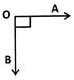QUESTION: 3

### What do we call an angle whose measurement is exactly equal to 0o?

Solution:
QUESTION: 4

What is an angle which measures exactly 180o called?

Solution:
QUESTION: 5

Which instrument is used to measure or construct angles?

Solution:

A protractor is a measuring instrument, typically made of transparent plastic or glass, for measuring angles. Most protractors measure angles in degrees (°). Radian-scale protractors measure angles in radians.

QUESTION: 6

How many rays can be drawn from a given point?

Solution:

An infinite number of rays can be drawn from a point.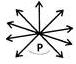QUESTION: 7

What do we call a 169o angle?

Solution:

An angle which lies between 90and 180o  is called as an obtuse angle. So, 169o  is an obtuse angle.

QUESTION: 8

What happens to the measurement of an angle after the extension of its arms?

Solution:

Extending the arms of an angle does not affect the angle between them.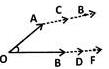QUESTION: 9

In ∠ROP, what is the vertex?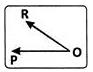Solution:

The vertex of an angle is the common point of the rays that form the arms of an angle. Here, it is O.

QUESTION: 10

What are the two arms of ZDEF?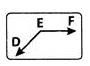Solution: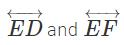are the two arms of ∠DEF.

QUESTION: 11

When two line segments meet at a point forming right angles, what type of segments are they called?

Solution:

Perpendicular segments meet at a point forming right angles.

QUESTION: 12

How is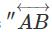is perpendicular to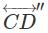written symbolically?

Solution:
QUESTION: 13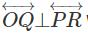What is the measure of ∠QOR ?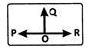Solution:

The correct option is Option C.

If OQ is perpendicular on PR, it means it forms right angles on both the sides.

∠QOP = ∠QOR = 180° ÷ 2 = 90°

QUESTION: 14

A line AB is parallel to the line CD. How is this symbolically written?

Solution:
QUESTION: 15

What are the lines which lie on the same plane and do not intersect at any point called?

Solution:

Since parallel lines are the lines which have equal distance throughout. So they never meet because they have constant distance between them.

QUESTION: 16

When two lines are parallel, what is the distance between them?

Solution:

The distance between the parallel  lines is the same.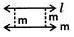QUESTION: 17

What is the number of pairs of parallel lines in the given figure?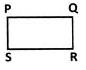Solution:

There are two pairs of parallel lines as the opposite sides of a rectangle are parallel.

QUESTION: 18

What is the complementary angle of 20o?

Solution:

Complementary angles add up to 90o . ∠PQR = 20⇒  Its complementary angle is 90o−20= 70o

QUESTION: 19

What is the supplementary angle of 120o?

Solution:

The sum of supplementary angles is 180o. ∠ABC = 120o ⇒ Its supplementary angles add up to 180o.

QUESTION: 20

What is the measure of a comple-mentary angle of an angle greater than 45o?

Solution:

Since the complementary angle of 45° is 45°, if the angle is greater than 45° its complement will be lesser than 45°.